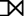# DBMS Joins: Inner, THETA, Outer, Equi Types of Join Operations

## What is Join in DBMS?

Join in DBMS is a binary operation which allows you to combine join product and selection in one single statement. The goal of creating a join condition is that it helps you to combine the data from two or more DBMS tables. The tables in DBMS are associated using the primary key and foreign keys.

In this DBMS tutorial, you will learn:

## Types of Join

There are mainly two types of joins in DBMS:

1. Inner Joins: Theta, Natural, EQUI
2. Outer Join: Left, Right, Full

Let’s see them in detail:

## Inner Join

Inner Join is used to return rows from both tables which satisfy the given condition. It is the most widely used join operation and can be considered as a default join-type

An Inner join or equijoin is a comparator-based join which uses equality comparisons in the join-predicate. However, if you use other comparison operators like “>” it can’t be called equijoin.

Inner Join further divided into three subtypes:

• Theta join
• Natural join
• EQUI join

## Theta Join

Theta Join allows you to merge two tables based on the condition represented by theta. Theta joins work for all comparison operators. It is denoted by symbol θ. The general case of JOIN operation is called a Theta join.

Syntax:

`A ⋈θ B`

Theta join can use any conditions in the selection criteria.

Consider the following tables.

 Table A Table B column 1 column 2 column 1 column 2 1 1 1 1 1 2 1 3

For example:

`A ⋈ A.column 2 >  B.column 2 (B)`
A ⋈ A.column 2 > B.column 2 (B)
column 1 column 2
1 2

## EQUI Join

EQUI Join is done when a Theta join uses only the equivalence condition. EQUI join is the most difficult operation to implement efficiently in an RDBMS, and one reason why RDBMS have essential performance problems.

For example:

`A ⋈ A.column 2 =  B.column 2 (B)`
A ⋈ A.column 2 = B.column 2 (B)
column 1 column 2
1 1

## Natural Join (⋈)

Natural Join does not utilize any of the comparison operators. In this type of join, the attributes should have the same name and domain. In Natural Join, there should be at least one common attribute between two relations.

It performs selection forming equality on those attributes which appear in both relations and eliminates the duplicate attributes.

Example:
Consider the following two tables

C
Num Square
2 4
3 9
D
Num Cube
2 8
3 18
`C ⋈ D`
C ⋈ D
Num Square Cube
2 4 8
3 9 18

## Outer Join

An Outer Join doesn’t require each record in the two join tables to have a matching record. In this type of join, the table retains each record even if no other matching record exists.

Three types of Outer Joins are:

• Left Outer Join
• Right Outer Join
• Full Outer Join

## Left Outer Join (AB)

Left Outer Join returns all the rows from the table on the left even if no matching rows have been found in the table on the right. When no matching record is found in the table on the right, NULL is returned.Consider the following 2 Tables

A
Num Square
2 4
3 9
4 16
B
Num Cube
2 8
3 18
5 75
`AB`
A ⋈ B
Num Square Cube
2 4 8
3 9 18
4 16

## Right Outer Join ( AB )

Right Outer Join returns all the columns from the table on the right even if no matching rows have been found in the table on the left. Where no matches have been found in the table on the left, NULL is returned. RIGHT outer JOIN is the opposite of LEFT JOIN

In our example, let’s assume that you need to get the names of members and movies rented by them. Now we have a new member who has not rented any movie yet.`AB`
A ⋈ B
Num Cube Square
2 8 4
3 18 9
5 75

## Full Outer Join ( AB)

In a Full Outer Join , all tuples from both relations are included in the result, irrespective of the matching condition.

Example:

`AB`
A ⋈ B
Num Square Cube
2 4 8
3 9 18
4 16
5 75

## Summary:

• There are mainly two types of joins in DBMS 1) Inner Join 2) Outer Join
• An inner join is the widely used join operation and can be considered as a default join-type.
• Inner Join is further divided into three subtypes: 1) Theta join 2) Natural join 3) EQUI join
• Theta Join allows you to merge two tables based on the condition represented by theta
• When a theta join uses only equivalence condition, it becomes an equi join.
• Natural join does not utilize any of the comparison operators.
• An outer join doesn’t require each record in the two join tables to have a matching record.
• Outer Join is further divided into three subtypes are: 1)Left Outer Join 2) Right Outer Join 3) Full Outer Join
• The LEFT Outer Join returns all the rows from the table on the left, even if no matching rows have been found in the table on the right.
• The RIGHT Outer Join returns all the columns from the table on the right, even if no matching rows have been found in the table on the left.
• In a full outer join, all tuples from both relations are included in the result, irrespective of the matching condition.